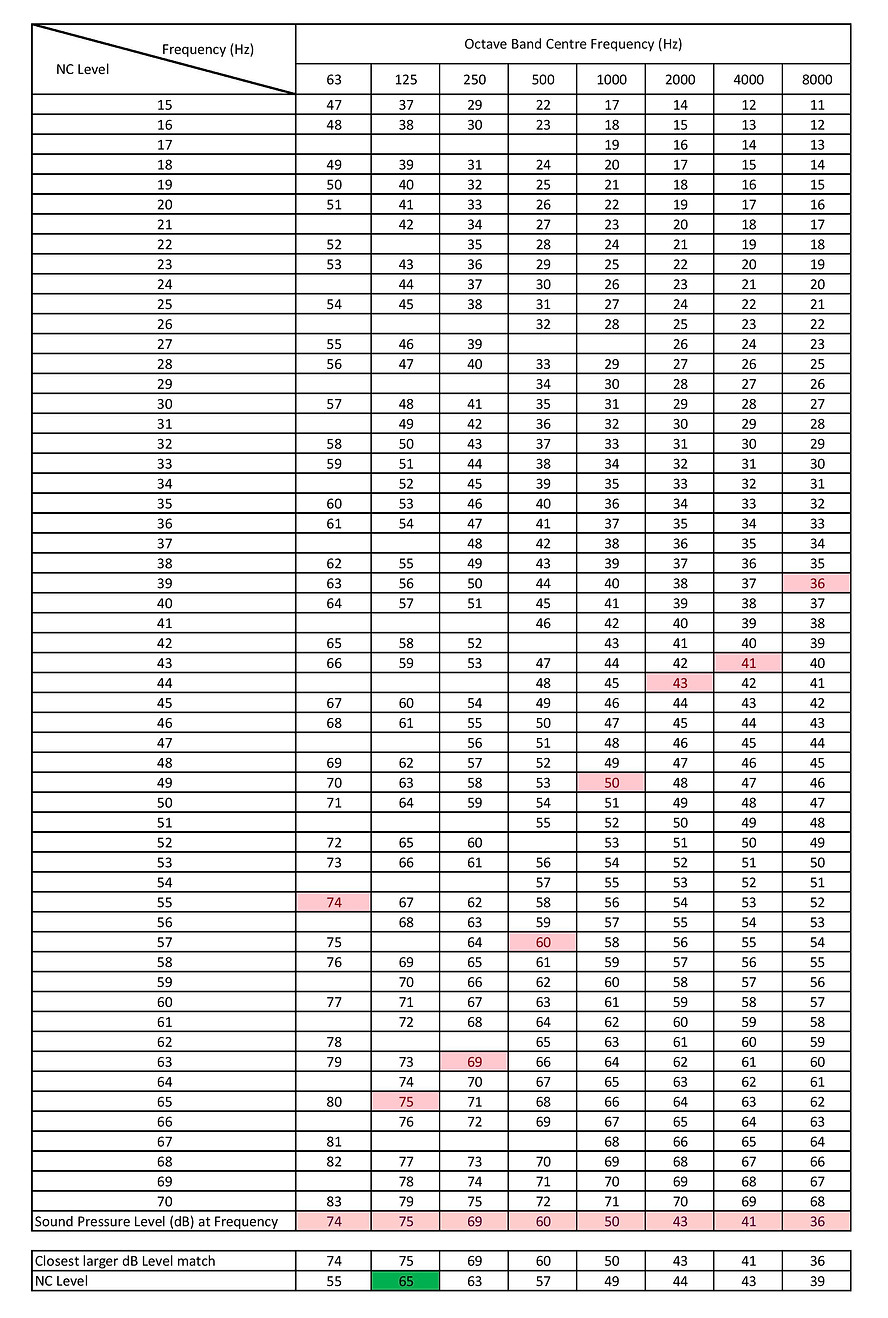top of page

NC and NR Level Calculator

Background noise arising from unoccupied spaces, or from the surrounding of the building – such as the air-conditioning system, traffic noise etc. – not only causes annoyance, stress and fatigue to the occupants, but also reduces productivity, concentration, hence to a certain extent, compromising safety. In severe cases, it even imparts ability to communicate.

Therefore, different methodologies have been developed to quantify those noises, so that they can be universally comparable, and can be set to comply to different sets of standards that govern noise levels for different applications.

NC (Noise Criterion) and NR (Noise Rating) are 2 ways of describing measured noise level across a frequency spectrum from, 63.5Hz to 8kHz. They are useful in determining noise levels attributable to mechanical systems such as motors, in noise-sensitive environments such as hotels and hospitals.

NC and NR are 2 combined representations of different noise levels (Octave Band Level) measured in dB across the octave band spectra at 63.5Hz, 125Hz, 250Hz, 500Hz, 1000Hz, 2000Hz, 4000Hz, and 8000Hz.

Definitions of NC and NR are being discussed below. Generally, NC curves are developed by the American National Standards Institute (ANSI). It is more commonly used in the United States, while NR curves are developed by the International Organization for Standardization (ISO). It is commonly used in the Europe.

Noise Criterion

Noise Criterion or NC Curves were established in the US for rating indoor noise such as noise from air-conditioning equipment etc. The NC rating of a spectrum is designated as the lowest NC curve above the measured octave-band spectrum.

The criterion defines the upper limits of each of the octave band spectra that must not be exceeded.NC Data Table (Downloadable in Excel File attached at the end of page)Worked Example

The following sound pressure levels are measured at the octave band frequencies below. Determine the NC level from the available data.These values can be compared to those in the NC data table and a value obtained for each octave band.From the table above, the NC level is taken as the highest value (highlighted green) among individual frequency bands, each of which is obtained by taking the equal (or closest larger) match of the sound pressure level (dB) measured from the test environment.

The NC value is the lowest NC curve whose sound pressure level is not exceeded in each individual frequency band. This can be shown by plotting the above NC data tabulation in the chart as shown below. From the Graph below, the lowest NC curve is NC 65, at which none of the frequencies of this level is exceeded by the measured sound pressure level.The NC value is the lowest NC curve whose sound pressure level is not exceeded in each individual frequency band.

The highest of these is the 125Hz band with an NC value of 65. Therefore the overall NC for this measurement is NC 65.Worked Example

The following sound pressure levels are measured at the octave band frequencies below. Determine the NC level from the available data.

The NR Curves

Noise Rating is graphical method of assigning a single number rating – i.e. the maximum acceptable noise level – to each of the octave band in the noise frequency spectrum. NR curves are in accordance with the International Organization for Standardization (ISO 1996) which was originally proposed to describe and determine the acceptable indoor environment for hearing preservation, speech communication and annoyance. However, NR is also widely used today to quantify noises from air-conditioning and mechanical ventilation systems in buildings.

The NR of the spectrum corresponds to the value of the first NR contour that is entirely above the spectrum.

Some examples of NR values and their applications are:NR Data Table (Downloadable in Excel File attached at the end of page)Worked Example

The following sound pressure levels are measured at the octave band frequencies below. Determine the NR level from the available data.These values can be compared to those in the NR data table and a value obtained for each octave band.From the table above, the NR Rating is taken as the highest value (highlighted green) among individual frequency bands, each of which is obtained by taking the equal (or closest larger) match of the sound pressure level (dB) measured from the test environment.

The overall NR value is the lowest NR curve whose sound pressure level is not exceeded in each individual frequency band.

This can be shown by plotting the above NR data tabulation in the chart as shown below. From the Graph below, the lowest NR curve is NR 62, at which none of the frequencies of this level is exceeded by the measured sound pressure level.The NR value is the lowest NR curve whose sound pressure level is not exceeded in each individual frequency band.

The highest of these is the 250Hz band with an NR value of 62. Therefore, the overall NR for this measurement is NC 62.

For ease of understanding, all NC & NR tabulation, charts and calculation presented above are incorporated in a downloadable Excel File below.

NC & NR Level Calculator (Excel)

bottom of page